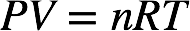# Problem: A 0.245-L flask contains 0.467 mol CO2 at 159 °C. Calculate the pressure:(a) using the ideal gas law

###### FREE Expert Solution

The ideal gas law is given by:where P = pressure in atm, V = volume in L, n = moles of gas, R = universal gas constant, and T = temperature in Kelvin.

We can use the ideal gas law to calculate the pressure of the gas. We're given:
V = 0.245 L          T = 159˚C + 273.15 = 432.15 K          n = 0.467 mol

Calculating for the pressure of the gas:

95% (203 ratings)###### Problem Details

A 0.245-L flask contains 0.467 mol CO2 at 159 °C. Calculate the pressure:

(a) using the ideal gas law